# On Impulsive Noise, CSP, and Correntropy

And I still don’t understand how a random variable with infinite variance can be a good model for anything physical. So there.

I’ve seen several published and pre-published (arXiv.org) technical papers over the past couple of years on the topic of cyclic correntropy (The Literature [R123-R127]). I first criticized such a paper ([R123]) here, but the substance of that review was about my problems with the presented mathematics, not impulsive noise and its effects on CSP. Since the papers keep coming, apparently, I’m going to put down some thoughts on impulsive noise and some evidence regarding simple means of mitigation in the context of CSP. Preview: I don’t think we need to go to the trouble of investigating cyclic correntropy as a means of salvaging CSP from the evil clutches of impulsive noise.

The various papers simply state that impulsive noise is a problem for cyclic methods of signal detection and modulation classification, but I don’t see any attempt to understand the root cause of the problem. And if you don’t know the root cause, you can’t very well rank the desirability of various solutions to the problem; you’re just treating your view of the symptoms, not the disease. In such cases, different people will resort to reaching for their favorite and trusted tools, not necessarily the best or simplest tool. Nails, hammers.

### What’s the Problem?: Impulsive Noise and its Effect on Blind Cycle-Frequency Estimation

First, what is impulsive noise? Generically, it is a signal with a probability distribution that is in some sense a heavy-tailed distribution (relative to, say, the Gaussian distribution). So most of the time it looks like typical Gaussian noise, but every once in a while there is a noise spike that is much larger than typical–it looks like an impulse.

The standard model for impulsive noise is alpha-stable noise, which is a random process that is governed by four parameters,$\alpha, \beta, \gamma$ and$\delta$ (sorry for the dual use of$\alpha$, this is not the same$\alpha$ that we usually use at the CSP Blog for cycle frequency). For some values of these parameters, the noise is actually Gaussian noise, but for others it is an impulsive noise whose second-order moment does not exist. [I’ve always been puzzled by using a mathematical model with infinite power to model observed noise in some system, which can’t have infinite power (can it?). Why not just restrict our attention to models that have heavy probability density function tails, but also finite but huge variances?]

MATLAB provides an alpha-stable noise generator as part of its random.m function. It takes the four basic distribution parameters as well as the size of the data structure that will contain draws from the distribution. A typical alpha-stable distribution corresponds to$\alpha = 1.5$$\beta = 0$,$\gamma = 1$, and$\delta = 0$:

[rv_vec_out] = random(‘stable’, alpha, beta, gamma, delta, [num 1]);

What happens is that the discrete-time alpha-stable impulsive-noise signal has a Gaussian-like component, but also possesses relatively rare high-amplitude samples–spikes or impulses. Here is a comparison between white Gaussian noise and alpha-stable impulsive noise using the typical parameters listed above:Figure 1. Time-domain views of Gaussian and alpha-stable impulsive noise. Note the rare but huge spikes in the latter.

Here the variance of each signal is equal to one. The rare large noise spikes are evident in the time-domain plot, and it is these spikes that cause trouble for blind cyclostationary signal processing algorithms. Why?

The main reason has to do with the form of the spectral coherence function. Recall that blind cycle-frequency estimation is efficiently and effectively done using a normalized version of the spectral correlation function called the coherence:$C_x^\alpha(f) = \displaystyle\frac{S_x^\alpha(f)}{[S_x^0(f+\alpha/2)S_x^0(f-\alpha/2)]^{1/2}}. \hfill (1)$

where$\displaystyle S_x^\alpha(f)$ is the spectral correlation function, and$\displaystyle S_x^0(f)$ is the power spectral density. Consider a single impulse: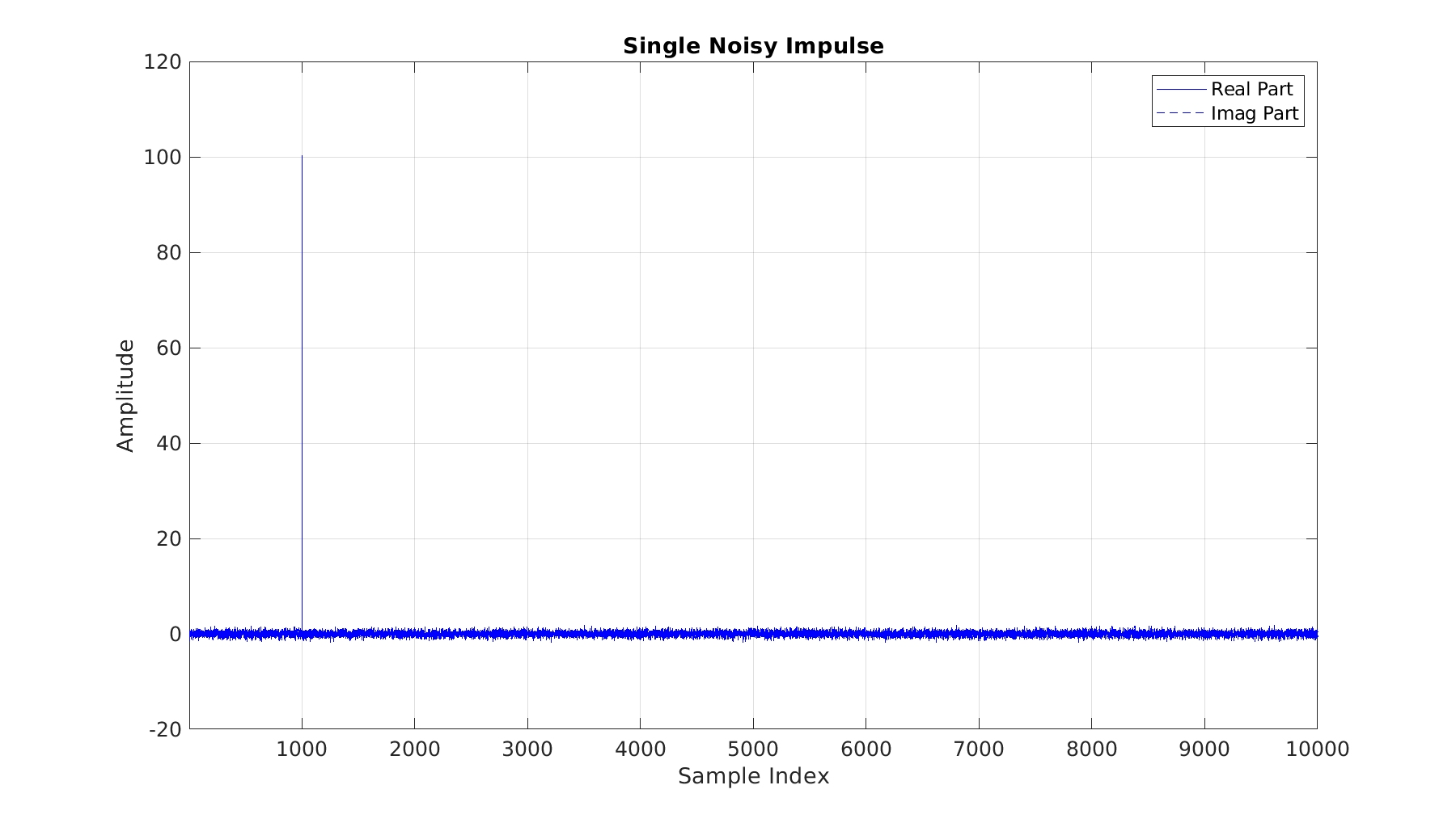Figure 2. Time-domain view of a single impulse in noise. The impulse occurs at sample 1000.

In either continuous time or discrete time, the Fourier transform of an impulse is a constant across frequency. Over time, if we correlate the various Fourier bins, we will see that they contain redundant information. That is, a single impulse has a form of spectral correlation! When we then form the coherence, we observe a very large number of high-coherence cycle frequencies–pretty much every possible cycle frequency has a high coherence.  Here is a plot of the blindly detected cycle frequencies for the noisy impulse shown above:Figure 3. Blindly estimated cycle frequencies for the data shown in Figure 2. Here the coherence is computed for all frequencies and cycle frequencies in the principal domain using spacings consistent with the measurement’s spectral and cycle-frequency resolutions.

The alpha-stable impulsive-noise signal is low-level more-or-less-normal noise plus randomly placed and scaled impulses, so it leads to a similar set of blindly detected cycle frequencies: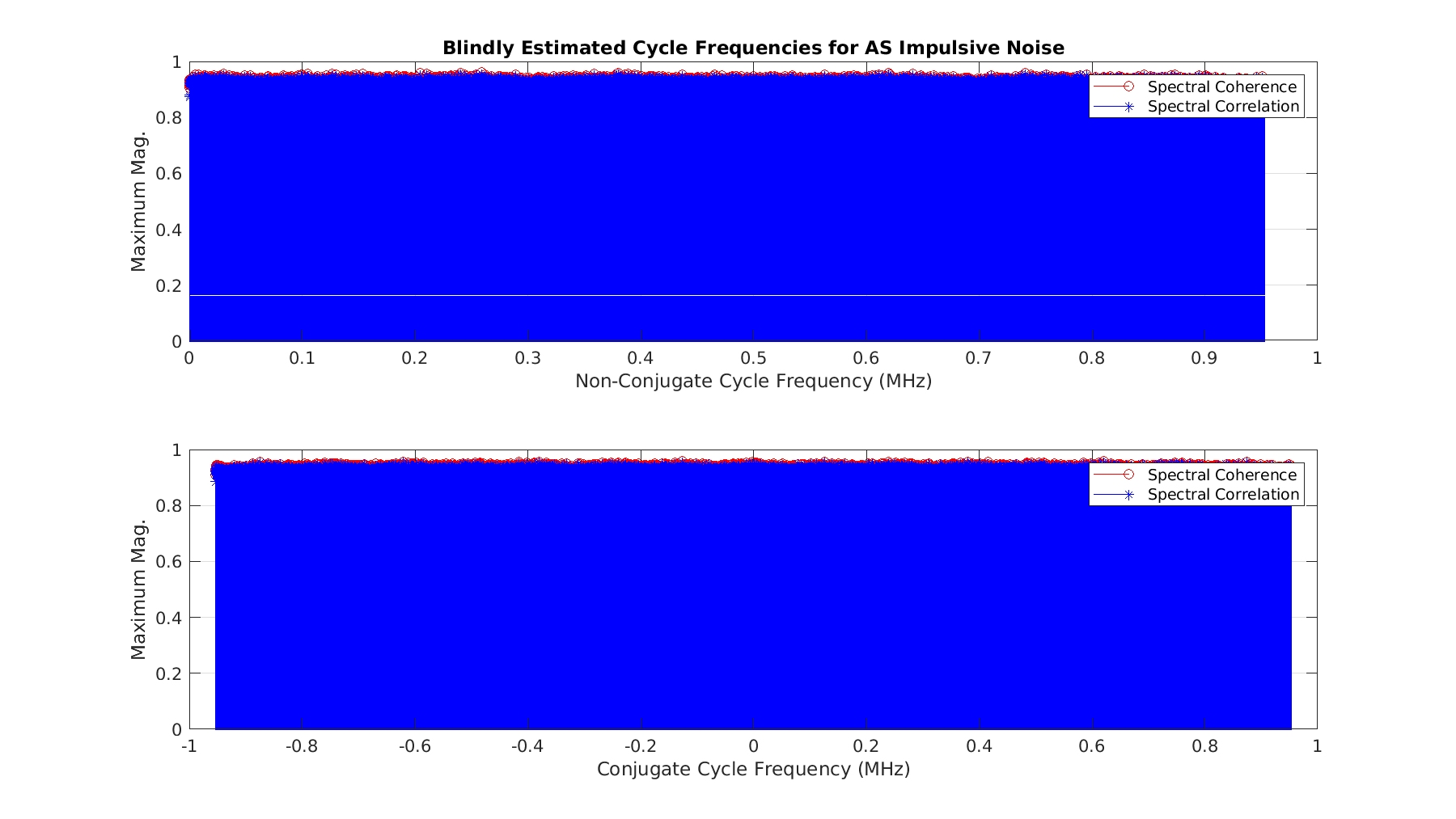Figure 4. Blindly estimated cycle frequencies for the alpha-stable impulsive noise shown in Figure 1.

The problem with impulsive noise with respect to blind CSP is that a great many false cycle frequencies are detected. If a modulated signal is also present, its cycle frequencies can be detected too, but the large number of other detected cycle frequencies makes recognizing the true (useful!) cycle frequencies difficult; they become lost in the crowd.

Here is an example using our old friend the textbook rectangular-pulse BPSK signal with symbol rate$100$ kHz and carrier offset frequency of$50$ kHz in additive white Gaussian noise (using the SSCA):Figure 5. Blindly estimated cycle frequencies for a rectangular-pulse BPSK signal in additive white Gaussian noise. The signal has a bit rate of kHz and a carrier frequency of kHz.

The plot shows that all of the cycle frequencies that fall within the diamond-shaped principal domain of the discrete-time/discrete-frequency spectral correlation function are detected, and no others. (See the posts on rectangular-pulse BPSK here and here.) In other words, this is the desired result: we see all the true BPSK cycle frequencies, and no false-alarm cycle frequencies.

Here are the blindly detected cycle frequencies for that same signal in alpha-stable impulsive noise (again using the SSCA):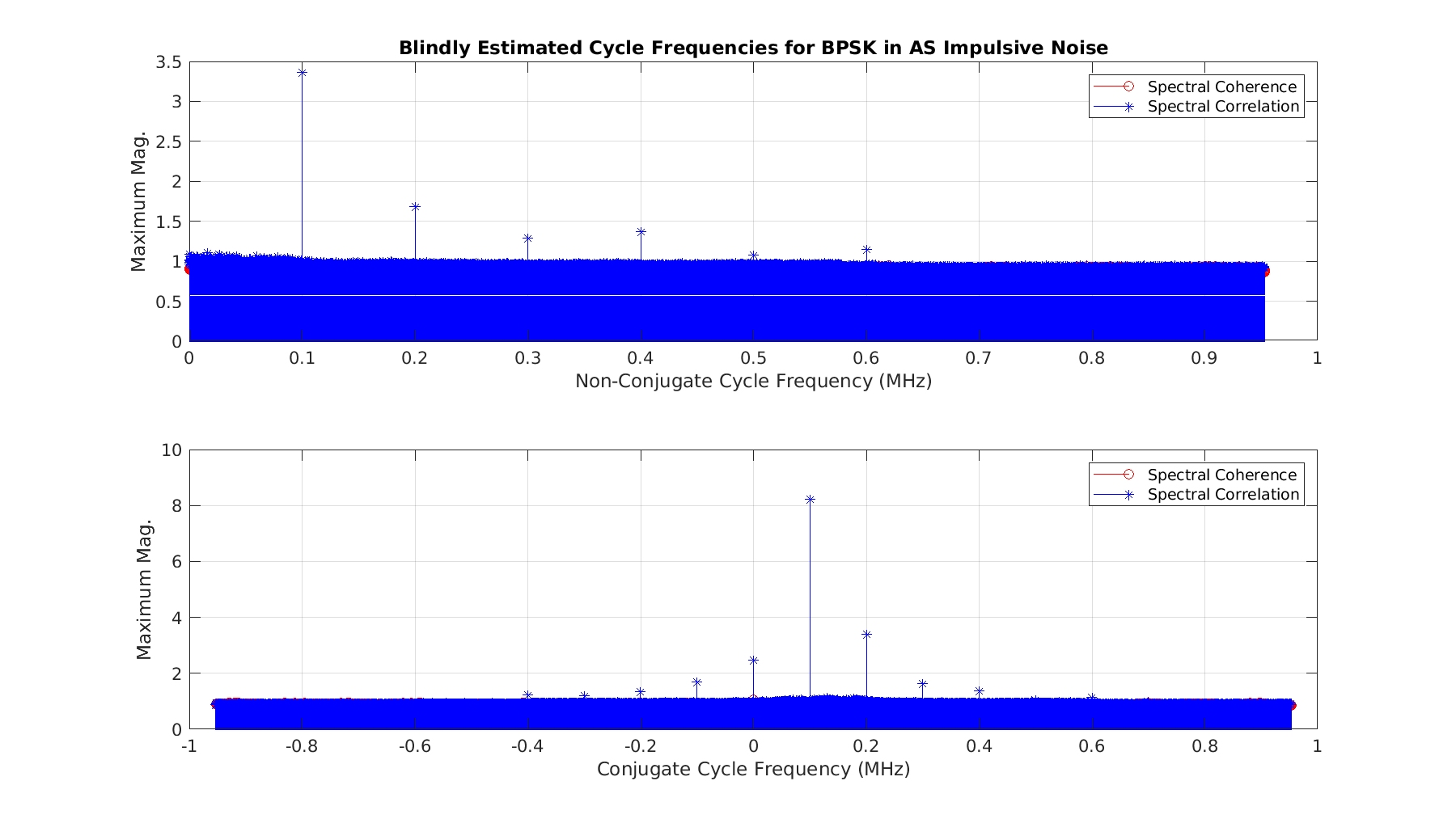Figure 6. Blindly estimated cycle frequencies for the rectangular-pulse BPSK signal used in Figure 5, but instead of embedding it in Gaussian noise, it is embedded in alpha-stable impulsive noise.

The BPSK cycle-frequency pattern is clear to our eyes, but an algorithm will be confronted with a much more difficult task of cycle-frequency identification/grouping than in the case of white Gaussian noise.  And think about what happens when the SNR decreases–even our eyes won’t find the pattern.

### Impulsive Noise and its Effect on Non-Blind SCF Estimation

In the non-blind case, we know the cycle frequencies of interest in advance of processing, and we apply some variant of the TSM or FSM to estimate the spectral correlation function, or perhaps the cyclic autocorrelation or spectral coherence functions. The effect of the alpha-stable impulsive noise on the resulting estimates is relatively minor. What follows is a sequence of spectral correlation and coherence plots for the BPSK signal used above, considering only the true cycle frequencies exhibited by the signal:Figure 7. Non-blindly estimated spectral correlation function for rectangular-pulse BPSK in Gaussian noise.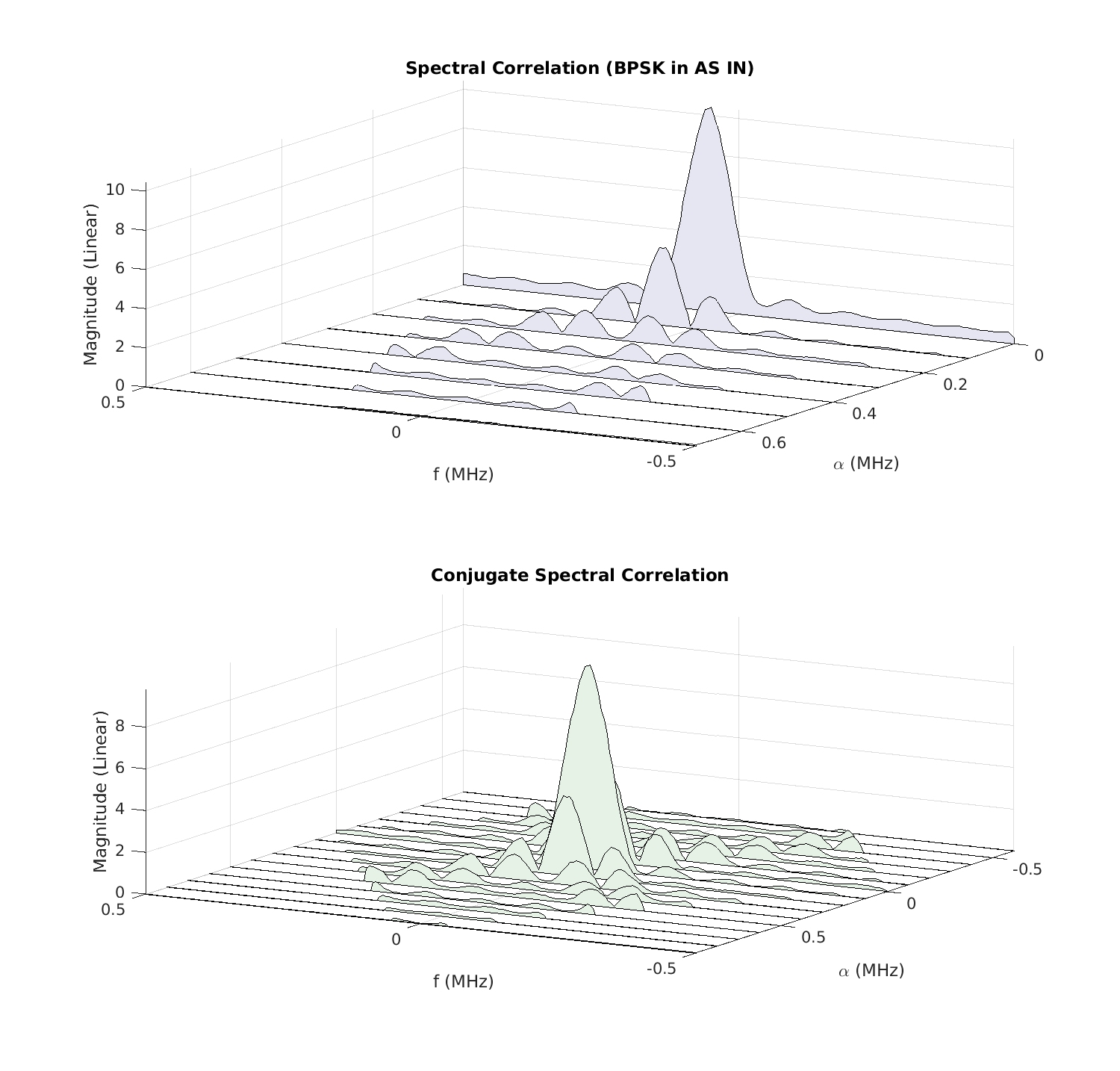Figure 8. Non-blindly estimated spectral correlation function for rectangular-pulse BPSK in alpha-stable impulsive noise.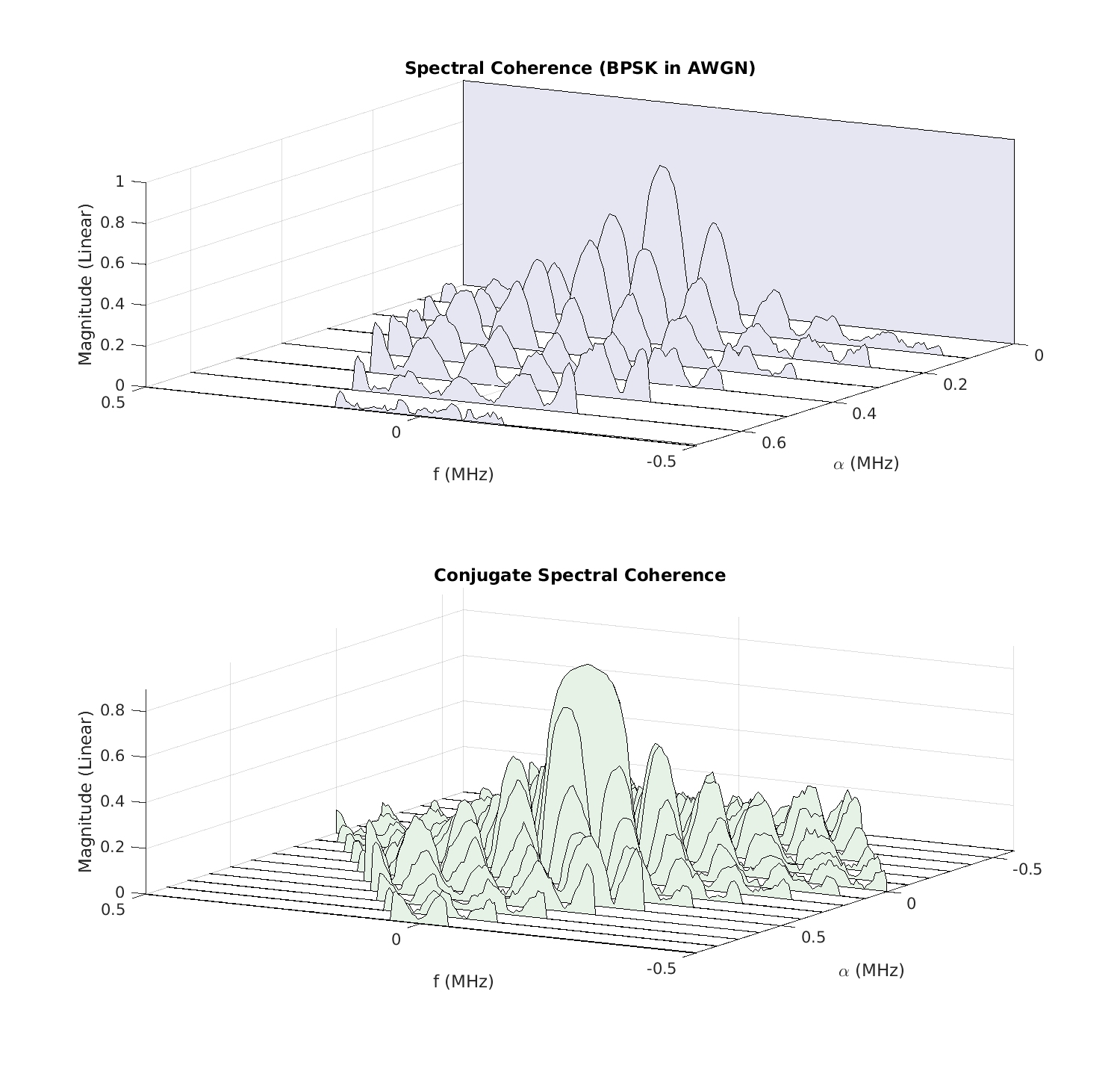Figure 9. Non-blindly estimated spectral coherence function for rectangular-pulse BPSK in Gaussian noise.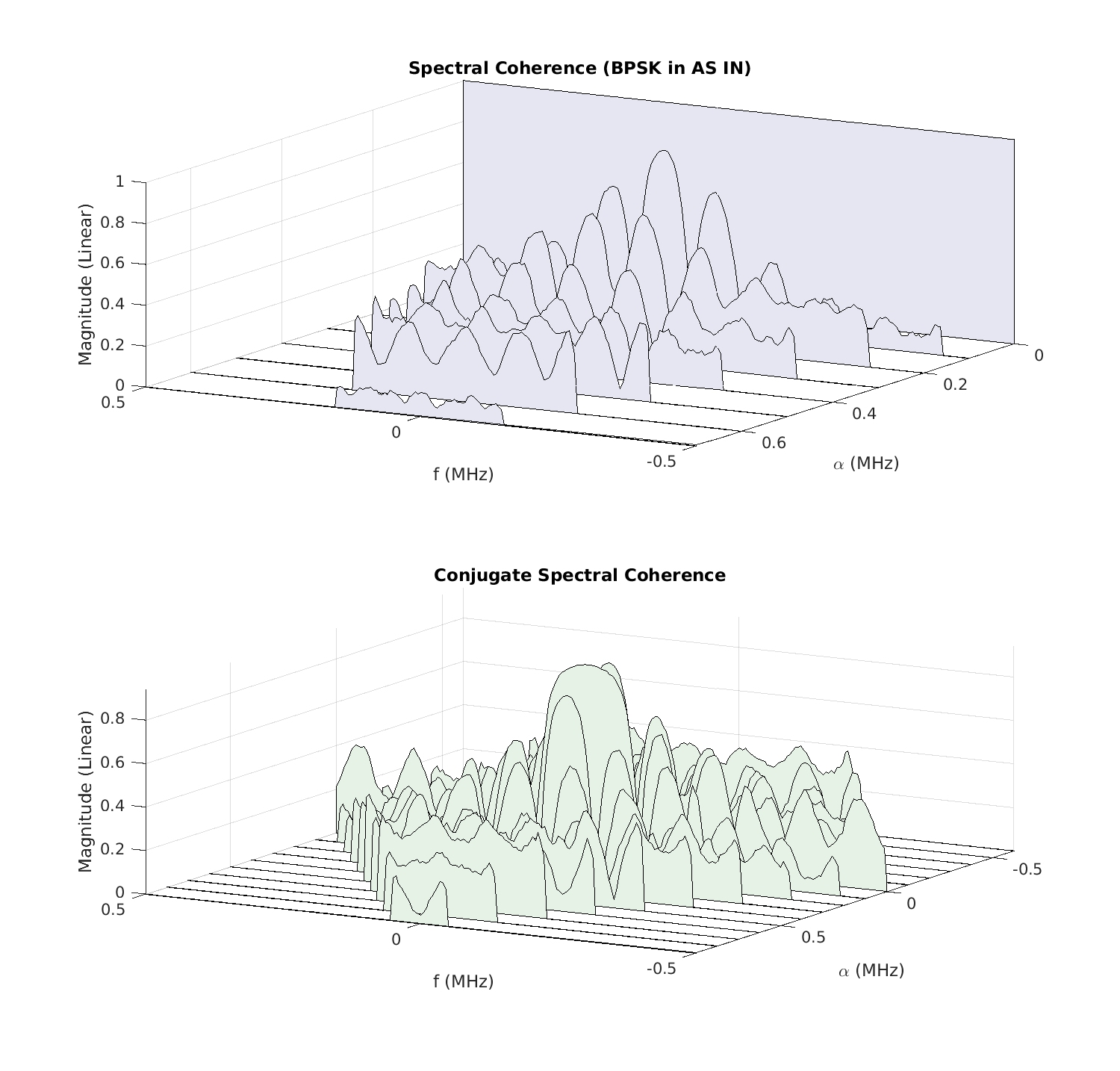Figure 10. Non-blindly estimated spectral coherence function for rectangular-pulse BPSK in alpha-stable impulsive noise.

### Mitigation Approaches

I suppose one mitigation approach is to develop cyclic correntropy, which is a nonlinear function of the data that essentially mixes together all possible cyclic moments of the input data. The kernel parameter controls the weighting that is placed on higher-order-moment components of the correntropy, so that one can obtain a result that is, for example, primarily a mixture of second- and fourth-order cyclic moments by selecting the appropriate kernel value.

One might apply some kind of signal-amplitude limiter so that large spikes are automatically reduced.

### My Preferred Approach

But why not attack the problem directly? Can we simply estimate the locations of the offending spikes and remove them? I think so.

First, to detect the locations of the larger of the spikes, we can apply a sorting routine to the incoming data-block samples’ magnitudes and declare the largest elements in the list as the spikes to be eliminated. More clearly, we can form a histogram of the magnitudes, determine the median of that histogram, and then declare all samples with magnitudes greater than$X$ times the median as spikes to be dealt with. So then we know the locations of the spikes. What next?

Two options come to mind. The first is to simply replace the spikes with zeros. The second is to replace the spike value by an interpolated value using the nearby non-spike values of the signal.

If the alpha-stable impulsive noise obeys the typical model, then the spikes are relatively rare (but still harmful), so replacing them with zero will not affect subsequent CSP estimators much. Somewhat better to fill the spikes in with interpolated values, and if only a few values are involved, the complexity is small.

So that’s what I do here: Find the median, fix$X$, find the locations of the spikes, and replace them with a simple interpolated value using the points on the left and the right of the spike.

### Processing Example: Blind Modulation Recognition and Parameter Estimation

Let’s see how this works on a blind modulation-recognition problem. I consider five input signal types: BPSK, QPSK,$\pi/4$-DQPSK, 16QAM, and MSK. The signals have unit power ($0$ dB), symbol rates of$0.2$ (normalized frequency), and carrier offsets that are chosen randomly from trial to trial on the interval$[-0.1, 0.1]$. The PSK and QAM signals use a bandwidth-efficient square-root raised-cosine pulse function with roll-off parameter$0.35$. The alpha-stable impulsive noise has a power equal to$15$ dB. The number of trials is$1000$ and the length of each processed noisy signal is$32,768$ samples.

I apply these signals to a blind modulation-recognition algorithm (see the ML Challenge post for more information) that makes extensive use of CSP, including the spectral correlation and coherence functions as well as high-order cyclic cumulants.

When I do no impulsive-noise mitigation, I obtain the following confusion matrix:Figure 11. Five-signal confusion matrix obtained by Monte Carlo simulation of a five-signal blind modulation-recognition problem. The input signals are corrupted by alpha-stable noise and no impulsive-noise mitigation is used prior to application of the CSP-based blind recognizer.

The decision called CF-CHAIN is a generic decision that happens when the system blindly detects the presence of a large number of harmonically related cycle frequencies, but cannot reconcile them with signals that produce such sets, such as direct-sequence spread spectrum signals. We can see that the presence of the strong impulsive noise ruins the ability of this algorithm to blindly find the signals’ cycle frequencies, and so there is no hope of performing the subsequent modulation recognition that is based on those cycle frequencies. (But an improved algorithm still might be able to do it.)

When I apply the median-based mitigation method as a preprocessor to the modulation-recognition algorithm, I get the following confusion matrix:Figure 12. Five-signal confusion matrix obtained by Monte Carlo simulation of a five-signal blind modulation-recognition problem. The input signals are corrupted by alpha-stable noise and a simple impulse detection and interpolation mitigator is used prior to application of the CSP-based blind recognizer.

### Concluding Remarks

I’m sure there are other ways of detecting and replacing the large noise spikes that are produced by alpha-stable impulsive-noise processes. The median-magnitude threshold-based method I outlined here applies to both blind and non-blind CSP, and greatly mitigates the deleterious effects of the impulses contained in impulsive noise. It is an effective pre-processor for CSP algorithms that are designed using benign noise models.

I’m wondering why the various papers that expressly state that CSP is highly vulnerable to impulsive noise don’t at least compare the correntropy machinery to relatively simple, straightforward impulsive-noise mitigation strategies such as that outlined here. Maybe correntropy is better! But there is a cost in using correntropy: The statistics of all orders are mixed together in the correntropy function. One of the key virtues of using cyclic cumulants is that the contributions to a detection/classification feature are separated in terms of cumulant order but also in terms of contributing signal. It is very easy to control exactly which orders of moments or cumulants are included in the processing. We’d like to retain that advantage while also mitigating the bad effects of the impulses.

Please consider leaving a comment, criticism, or correction in the Comments Section below.## Author: Chad Spooner

I'm a signal processing researcher specializing in cyclostationary signal processing (CSP) for communication signals. I hope to use this blog to help others with their cyclo-projects and to learn more about how CSP is being used and extended worldwide.

Posted on

## 3 thoughts on “On Impulsive Noise, CSP, and Correntropy”

1.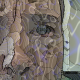jayprich says:

Does the impulse noise model have to be so sparse in the spatial domain? Surely many scenarios involve a more continuous crackle (additive) or intermittency (multiplicative) that is not so obvious to pre-process away.

1.Chad Spooner says:

Jayprich:

Thanks for the comment and for visiting the CSP Blog!

I’m not doing any spatial signal modeling or spatial filtering here. Perhaps you mean “time domain?”

I used the oft-cited alpha-stable model with the oft-used parameters, just to keep the criticism applicable to that portion of the literature that’s been visible to me. The authors I cited, and many more over the past 20 years or so, use that alpha-stable impulsive-noise model. It is so common (from my point of view!) that it is often just referred to as impulsive noise (like there is no other model of importance). The sparsity of the impulses in the time domain follows.

Can you point me, and my readers of course, to information sources that elaborate on the continuous crackle? I’m not sure it would qualify for the moniker “impulsive noise,” but I’d sure like to understand what you’re referring to.

2.jayprich says:

Thanks for replying, I stand corrected about the sparsity of alpha-stable extrema. Yes I meant temporal not spatial separation.

I don’t know what parameters are used in the literature for a Levy alpha-stable distributions. Listening to generated sound with alpha between 1.5 and 1.7 I hear mainly hiss with a hint of crackle .. admittedly my computer speaker cannot reproduce the loudest pops in the data.

I don’t have a specific reference but think Poisson (shot noise) or Bernoulli (binary noise with low probability) have been used as statistical models for impulse noise with finite variance.

I take your point that straightforward impulsive-noise mitigation may avoid artifacts in the cumulants.